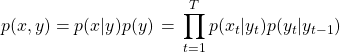## How do you generate text using a hMM model given below:One possible interpretation of the latent variables in the HMM model is that they are POS tags. We will go with this interpretation for simplicity, though the latent states could mean other things as well. To generate text from a HMM, we need to know the transition matrix (the probability of going from one tag…

## What order of Markov assumption does n-grams model make ?

An n-grams model makes order n-1 Markov assumption. This assumption implies: given the previous n-1 words, probability of  word is independent of words prior to words. Suppose we have k words in a sentence, their joint probability can be expressed as follows using chain rule:      Now, the Markov assumption can be used to make…

## How is long term dependency maintained while building a language model?

Language models can be built using the following popular methods – Using n-gram language model n-gram language models make assumption for the value of n. Larger the value of n, longer the dependency. One can refer to what is the significance of n-grams in a language model for further reading. Using hidden Markov Model(HMM) HMM maintains long…

## How to measure the performance of the language model ?

While building language model, we try to estimate the probability of the sentence or a document. Given sequences(sentences or documents) like     Language model(bigram language model) will be :     for each sequence given by above equation. Once we apply Maximum Likelihood Estimation(MLE), we should have a value for the term . Perplexity…

## What is a language model ? How do you create one ? Why do you need one ?

A language model is a probability distribution over sequences of words P(w_1,… ,w_m). It enables us to measure the relative likelihood of different phrases. Measuring the likelihood of a sequence of words is useful  in many NLP tasks such as speech recognition, machine translation, POS tagging, parsing, and so on. Example :  In any generative…

## How will you build an auto suggestion feature for a messaging app or google search?

Auto Suggestion feature involves recommending the next word in a sentence or a phrase. For this, we need to build a language model on large enough corpus of “relevant” data. There are 2 caveats here – large corpus because we need to cover almost every case. This is important for recall. relevant data is useful…